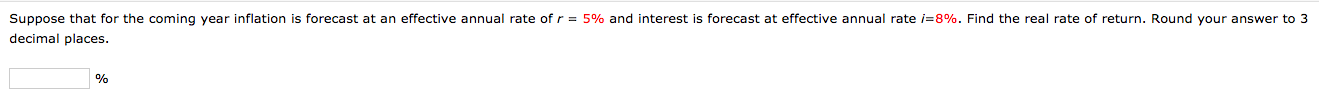Home / Answered Questions / Other / suppose-that-for-the-coming-year-inflation-is-forecast-at-an-effective-annual-rate-of-r-5-and-intere-aw698

# (Solved): Suppose That For The Coming Year Inflation Is Forecast At An Effective Annual Rate Of R = 5% And Int...Suppose that for the coming year inflation is forecast at an effective annual rate of r = 5% and interest is forecast at effective annual rate i=8%. Find the real rate of return. Round your answer to 3 decimal places.

We have an Answer from Expert